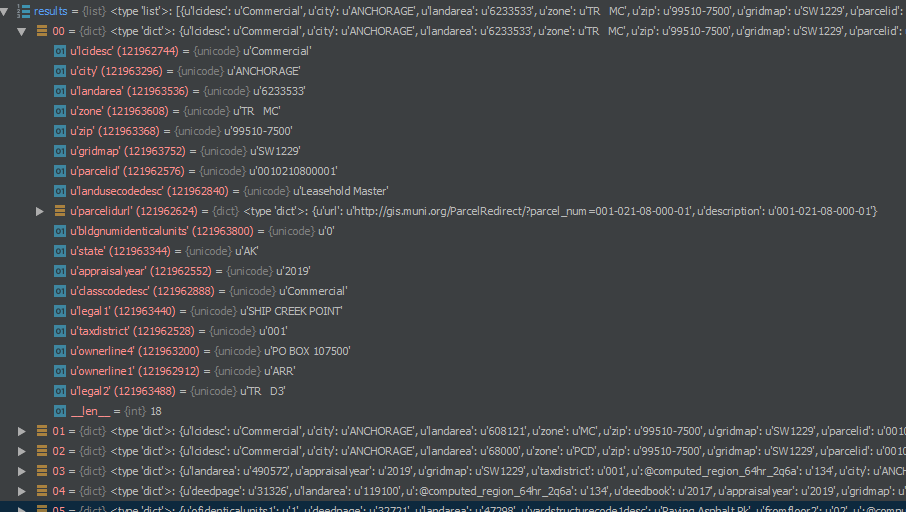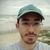# Pandas dataframe to Esri Table

8061
7
10-28-2019 04:21 PMbyMVP Regular Contributor

I have a pandas data frame (df) that I want to put into an Esri table in sde. I am having a hard time dealing with the datatypes in an effective way. All the columns in the df have the datatype object. So, after some digging, it looks like strings get the data-type object in pandas. There is a good explication for why this is on StackOverflow:

All, well and good. After I convert it to a numpy array the datatype is 'O' and then to an Esri table it fails. I think the problem is in the datatype. When I set the datatype to '|S256' it works fine. I also suspect that some of the columns have a data type of int or float.

So, I need an elegant way to detect the data type and assign it to the numpy array. Suggestions?

This is the code so far. It works fine. I am dropping down to one column for testing. But, there are around 250 columns between two tables in the real data.

```from sodapy import Socrata
import cama_helper

client = Socrata('data.muni.org', None)

if __name__ == '__main__':
client = Socrata('data.muni.org', None)
for cama_id, cama_name in {'r3di-nq2j': 'cama_residential', 'ijws-5rpw': 'cama_commercial'}.items():
df = cama_helper.get_data_as_panda(client, cama_id)
```

# cama_helper

import pandas
import arcpy
import numpy

def get_data_as_panda(client, cama_id):
results = client.get(cama_id, limit=20)
df = pandas.DataFrame.from_records(results)
df.drop(df.columns[[0, 1, 2]], axis=1, inplace=True)
return df

s = df.iloc[:, 1]
df = s.to_frame()
#numpy_array = df.to_records()
numpy_array = df.to_numpy()
numpy_array2 = numpy.array(numpy_array, numpy.dtype([('textfield', '|S256')]))
arcpy.da.NumPyArrayToTable(numpy_array2, r'Temp.gdb\{}'.format(cama_name))```
Tags (3)
7 RepliesbyMVP Legendary Contributor

If the pandas df contains fields of mixed dtypes, this will be reflected in the dtype of the resultant numpy array.

These are "structured arrays" (recarrays as similar but use a dot notation for field calling) .

From there you can use NumPyArrayToTable.

A sample array with mixed dtype showing a combination of integer, float and string (Unicode now) data

``````d # ---- a sample structured array
array([( 1, 2, 19, 0, 10,  0, 300004.72, 5000004.73, 0.9 , 'A', 'a'),
( 2, 2, 19, 0,  8,  5, 300017.5 , 5000007.  , 0.93, 'B', 'a'),
... snip
(12, 1, 13, 0,  9, 10, 300033.06, 5000025.98, 1.04, 'C', 'a')],
dtype=[('OID_', '<i4'), ('Parts', '<i4'), ('Points', '<i4'),
('Curves', '<i4'), ('Value_0', '<i4'), ('Sorted_', '<i4'),
('X_cent', '<f8'), ('Y_cent', '<f8'), ('Area_perim', '<f8'),
('A0', '<U5'), ('A1', '<U5')])

# --- i4 is np.int32
# --- f8 is np.float64
# --- U5  is unicode, 5 characters wide‍‍‍‍‍‍‍‍‍‍‍‍

# ---- pull out some data using name slicing

d['Points']
array([19, 19,  4,  5,  7, 30, 24,  7,  7,  4,  6, 13])‍‍‍‍‍‍‍‍‍‍‍‍‍‍‍‍‍‍``````

so pull in all your data to see if you have conventional single structured fields or nested fieldsbyMVP Regular Contributor

Thanks Dan. I get the structured array bit. The problem is that the source df from the city is all of the type object. So, it would be nice to detect the "true" data type of each column and assign that to the structured array. I guess I could do it by hand and then make a fixed schema to load the data into but with 270 columns this could be quite the task. Also, it would be nice to have the program be dynamic so that if the source schema changed the schema in sde could be made to reflect it.byMVP Legendary Contributor

a dtype of object is a tough one since there is no guarantee that any of the objects in the array have a common dtype, especially if it contains geometry.

Even if it an object dtype, and the first record can be read revealing the structured format of the rest of the records that would be too easy.  Since the whole array is an object array, I suspect that there may be more than one array/file in there if it is an *.npz format.

How are you getting the array?  (as a *.npy or *,npz file are best)

What is the shape of the whole object array ( arr.shape )?

the shape of the first record (arr.shape ) and the last arr[-1].shape

If you are comfortable working with numpy dissecting it should be an easy task.

I am just surprised that the city doesn't provide any metadatabyMVP Regular Contributor
a dtype of object is a tough one since there is no guarantee that any of the objects in the array have a common dtype, especially if it contains geometry.

So, most of the columns have string values, but some have date or integer values. So, would I need to iterate over each value in every row and test (for string, int, float, date) for each column to nail down the datatypes?

How are you getting the array?  (as a *.npy or *,npz file are best)

It starts as a list of dictionaries. Then I turn it into pandas and then into a numpy array. There does not appear to be any datatype information.The shape of the array in (20, ) which is weird. I would have thought it would (20, 130) or something. (keep in mind that I am only grabbing the first 20 rows of the dataset. The real thing has a few hundred thousand rows)

the shape for array and array[-1] are (). which also feels weird. I guess I don't understand shape in this case.byMVP Legendary Contributor

The journey of the array

Numpy structured array, to dataframe and back to a recarray.  The first and last are 'essentially the same'.  The dataframe is largely a numpy array in origins with added decorations.

``````d # ---- numpy structured array
array([( 1, 300004.72, 5000004.73, 2, 19, 0, 'A', 'a', 10, 0.9 ,  0),
( 2, 300017.5 , 5000007.  , 2, 19, 0, 'B', 'a',  8, 0.93,  5),
.... snip ....
(11, 300029.88, 5000011.35, 1,  6, 0, 'B', 'b',  8, 1.06,  8),
(12, 300033.06, 5000025.98, 1, 13, 0, 'C', 'a',  9, 1.04, 10)],
dtype=[('OID_', '<i4'), ('X_cent', '<f8'), ('Y_cent', '<f8'), ('Parts', '<i4'),
('Points', '<i4'), ('Curves', '<i4'), ('A0', '<U5'), ('A1', '<U5'),
('Value_0', '<i4'), ('Area_perim', '<f8'), ('srt_a0a1', '<i4')])

df = pd.DataFrame.from_records(d)  # ---- convert to a data frame

d0 = df.to_records()               # ---- convert the data frame back to an array

df
OID_         X_cent        Y_cent  Parts  ...  A1  Value_0 Area_perim srt_a0a1
0      1  300004.717949  5.000005e+06      2  ...   a       10   0.902025        0
1      2  300017.500000  5.000007e+06      2  ...   a        8   0.928571        5
2      3  300013.000000  5.000013e+06      1  ...   a        3   0.744316        1
.... snip ....
10    11  300029.876867  5.000011e+06      1  ...   b        8   1.057053        8
11    12  300033.056389  5.000026e+06      1  ...   a        9   1.036191       10

[12 rows x 11 columns]

d0
rec.array([( 0,  1, 300004.72, 5000004.73, 2, 19, 0, 'A', 'a', 10, 0.9 ,  0),
( 1,  2, 300017.5 , 5000007.  , 2, 19, 0, 'B', 'a',  8, 0.93,  5),
( 2,  3, 300013.  , 5000012.67, 1,  4, 0, 'A', 'a',  3, 0.74,  1),
.... snip ....
(10, 11, 300029.88, 5000011.35, 1,  6, 0, 'B', 'b',  8, 1.06,  8),
(11, 12, 300033.06, 5000025.98, 1, 13, 0, 'C', 'a',  9, 1.04, 10)],
dtype=[('index', '<i8'), ('OID_', '<i4'), ('X_cent', '<f8'), ('Y_cent', '<f8'),
('Parts', '<i4'), ('Points', '<i4'), ('Curves', '<i4'), ('A0', 'O'),
('A1', 'O'), ('Value_0', '<i4'), ('Area_perim', '<f8'),
('srt_a0a1', '<i4')])
‍‍‍‍‍‍‍‍‍‍‍‍‍‍‍‍‍‍‍‍‍‍‍‍‍‍‍‍‍‍‍‍‍‍‍‍‍‍‍‍‍‍‍‍‍‍‍‍‍‍‍‍‍‍‍‍‍‍‍‍‍‍‍‍‍‍‍‍‍‍‍‍‍‍‍‍‍‍‍‍‍‍‍‍‍‍‍‍‍‍‍‍‍‍‍‍‍‍‍‍‍‍‍``````byMVP Regular Contributor

Thanks Dan. I think I am just going to stop fighting it and stuff everything into Oracle as strings. Then the applications on the other side can add validation as needed.by
New Contributor III

I've been able to import a pandas dataframe using arcgis.features.GeoAccessor.to_table . It doesn't require a geometry if you are importing to a table (as opposed to GeoAccessor.to_featureclass which requires a spatially-enabled dataframe)

``df.spatial.to_table(location=location)``